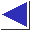# comp.lang.c FAQ list ·Question 13.21

Q: I'm porting this program, and it calls a routine drand48, which my library doesn't have. What is it?

A: drand48 is a Unix System V routine which returns floating point random numbers (presumably with 48 bits of precision) in the half-open interval [0, 1)[footnote] . (Its companion seed routine is srand48; neither is in the C Standard.) It's easy to write a low-precision replacement:

```#include <stdlib.h>

double drand48()
{
return rand() / (RAND_MAX + 1.);
}
```

To more accurately simulate drand48's semantics, you can try to give it closer to 48 bits worth of precision:

```#define PRECISION 2.82e14	/* 2**48, rounded up */

double drand48()
{
double x = 0;
double denom = RAND_MAX + 1.;
double need;

for(need = PRECISION; need > 1;
need /= (RAND_MAX + 1.)) {
x += rand() / denom;
denom *= RAND_MAX + 1.;
}

return x;
}
```

Before using code like this, though, beware that it is numerically suspect, particularly if (as is usually the case) the period of rand is on the order of RAND_MAX. (If you have a longer-period random number generator available, such as BSD random, definitely use it when simulating drand48.)

References: PCS Sec. 11 p. 149